# Construction of a Line Parallel to a Given Line, Through a Point Not on the Line:

Let us begin with an activity

(i) Take a sheet of paper. Make a fold. This fold represents a line l.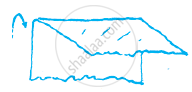(ii) Unfold the paper. Mark a point A on the paper outside l.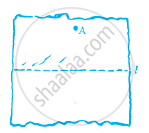(iii) Fold the paper perpendicular to the line such that this perpendicular passes through A. Name the perpendicular AN.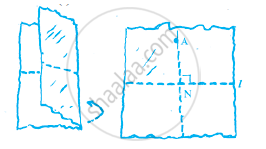(iv) Make a fold perpendicular to this perpendicular through point A. Name the new perpendicular line as m. Now, l || m.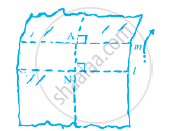## 1. Construction using ruler and compasses only:

Step 1: Take a line 'l' and a point 'A' outside 'l'.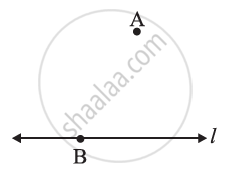Step 2: Take any point B on l and join B to A.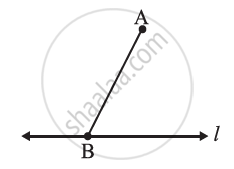Step 3: With B as centre and a convenient radius, draw an arc cutting l at C and BA at D.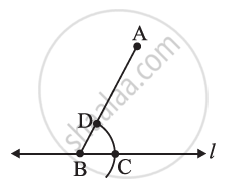Step 4: Now with A as a centre and the same radius as in Step 3, draw an arc EF cutting AB at G.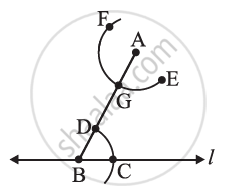Step 5: Place the pointed tip of the compasses at C and adjust the opening so that the pencil tip is at D.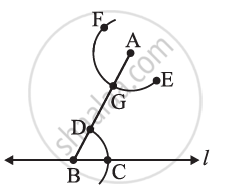Step 6: With the same opening as in Step 5 and with G as the centre, draw an arc cutting the arc EF at H.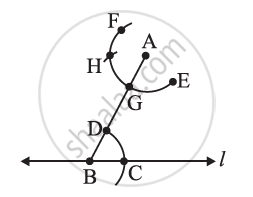Step 7: Now, join AH to draw a line 'm'.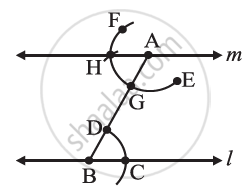Note that ∠ABC and ∠BAH are alternate interior angles. Therefore m || l.

If you would like to contribute notes or other learning material, please submit them using the button below.

### Shaalaa.com

Parallel Lines by Corresponding Angles [00:03:00]
S
0%